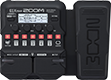# Where the streets have no name

Discussion in 'Zoom G1/G1X Four' started by Metalvalak, Oct 22, 2020.

1. Where the streets have no nameDevice: Zoom G1X Four
Firmware: 2.00

Name on device: Streets
Optimized for: Phones/Speaker

Effects chain:For stratocaster, second position pickup, use textured pick for better sound

Effect: "Comp" (Dynamics), active - "yes"
"Sense" = 3
"Attack" = Fast
"Tone" = 5
"Volume" = 80

Effect: "MATCH30" (Amp simulator), active - "yes"
"Gain 1" = 11
"Bass 1" = 47
"Treble 1" = 49
"Gain 2" = OFF
"Tone 2" = 1
"Cut" = 0
"Volume" = 80

Effect: "Delay" (Delay), active - "yes"
"Time" = 350
"F.B." = 26
"Mix" = 70
"Tail" = Off

Effect: "Gt GEQ 7" (Filter), active - "yes"
"100" = 5.5
"200" = 0.5
"400" = 2.5
"800" = 2.0
"1.6k" = 8.0
"3.2k" = 7.5
"6.4k" = 7.0
"VOL" = 56

Effect: "Hall" (Reverb), active - "yes"
"PreD" = 49
"Decay" = 10
"Mix" = 46
"Tail" = Off

Patch Volume: 100